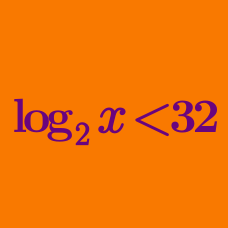Algebra

# Logarithmic Inequalities: Level 4 Challenges

$\log_{4} x^2\ + \log_{x} 16\ - 5 < 0$

How many positive integer solutions can satisfy the inequality above?

$\log _{ 3 }{ \frac { \left| { x }^{ 2 }-4x \right| +3 }{ { x }^{ 2 }+\left| x-5 \right| } } \ge 0$

Solve the following for $x$.

###### Try Fun with inequalities-1 and Fun with inequalities-2

$\log_{5n} 30\sqrt{5} \ge \log_{4n} 48$

Over the domain $n > 1$, let $M$ be the smallest value of $n$ that satisfies the above inequality.

What is $M^3?$

Given that

$\begin{array} {lll} 0.161 & < \log_x 2 < & 0.162, \\ 0.256 & < \log_x 3 < & 0.257, \\ 0.375 & < \log_x 5 < & 0.376,\\ \end{array}$

determine $\lfloor \log_x 7^{100} \rfloor$.

$\ln { (x^{ 2 }+\sqrt { 2 } x+1) } \le \ln { (x^{ 4 }+1) }$

find the minimum positive value that $x$ can have that satisfies the inequality above

write your answer as the square of the number

×

Problem Loading...

Note Loading...

Set Loading...